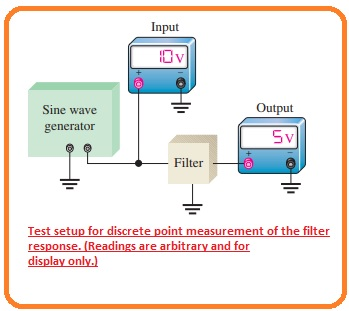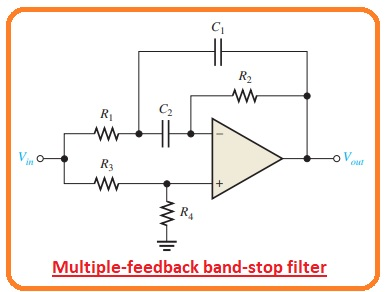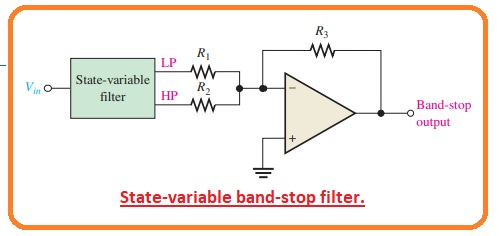High friends, I hope you all doing great. In today’s post, we will have a detailed look at Two Methods for Measuring Frequency Response. The main techniques for measuring the filter response of frequency measurements are discrete point measurement and swept frequency measurements.

In this post, we will discuss these 2 methods one by one with the detail and discuss their circuit and other related parameters. So let’s get started with Discuss Two Methods for Measuring Frequency Response.

#### Discrete Point Measurement

• Below the figure, you can see the circuit configuration for getting the filter output voltage calculations at different values of input frequency value with the general usage of any measuring device.
• The process is described here with the details.• Adjust the amplitude of the sinusoidal signal generator at the required voltage level.
• Adjust the frequency of the sinusoidal signal generator at a level that is less than the required critical frequency of filter under observation. In case of low pass filter adjust the frequency close to zero hertz. in case of bandpass filter adjust the frequency less than the required lower critical frequency.
• Increase the value of frequency in already adjusted steps to permit certain information for the correct response curve.
• Retain the constant value of input single amplitude and change the value of frequency.
• Measure every value of output voltage at each value of frequency.
• After measuring the specific values make a graphical representation of output voltage with the frequency.

#### Swept Frequency Measurement

• This technique needed further elaboration testing devices than the previous technique, but this technique is efficient than the discrete point and provides correct response curvature.
• The circuit for this technique is shown in the below figure. In which swept frequency generator and spectrum analyzer is used.
• diagram denoted as b indicates that how testing can be performed with the usage of the oscilloscope.
• The swept-frequency generator generates a constant amplitude output signal whose frequency rises in a linear way among the 2 preset restrictions.
• The spectrum analyzer is compulsory to elaborate oscilloscope which can be measured for a required frequency division rather than the general time setting.
• So input frequency at the filter sweeps in the already adjusted range the response curvature is located at the display of spectrum analyzer.#### Multiple-Feedback Band-Stop Filter

• Below figure indicate the circuit configuration of multiple feedback bandstop filter.
• Note that this arrangement i like the bandpass category which we discussed in previous post with the difference is that resistance R3 has changed its position and R4 has inserted.#### State-Variable Band-Stop Filter

• adding the low pass and high pass responses obtained with the state variable filter to the summing amplifier makes a band stop filter can be seen in the below figure.
• The main usage of this category of the filter is reducing the sixty-hertz frequency hun in audio networking with the adjustment of the center frequency to six.That is all about Two Methods for Measuring Frequency Response I tried my level best to make it simple for you if you have any further query ask in comments. Thanks for reading. Have a good day